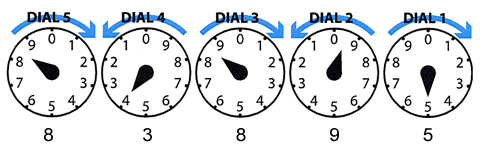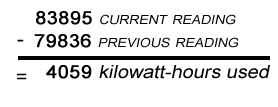Read all the dials from right to left, and write the numbers down from right to left. When the hand on any dial is between two numbers, read the smaller number.

If the pointer appears to be exactly on a number, you should record the next lowest number UNLESS the pointer on the dial to its right has passed zero. Dial 1 has nothing on its right, so if the dial 1 pointer is precisely on a number, record that number.In the above diagram, the reading is 83895.

### Step-by-step instructions

• Dial 1: The pointer is directly on number 5. Record as 5, since there's no dial to its right.
• Dial 2: The pointer has just passed 9, and is between 9 and 0. Record as 9.
• Dial 3: The next dial has passed 8, and is between 8 and 9. Again, record the smaller number which is 8.
• Dial 4: The pointer looks like it is right on the 4, but the dial to its right has not passed zero. So, you would record this dial as 3.
• Dial 5: The pointer is between 8 and 9. Record the smaller number which the pointer just passed, which is 8.

Subtract the previous month's reading from the current reading to find out how much electricity you used since your meter was last read.Note: Many meters in homes or apartments have four dials rather than five. Read these meters the same way.

### Still confused?

• Notice that the pointers on the first, third and fifth dial move in the same directions as the hand of a clock. The second and fourth pointers move in the opposite direction.
• Stand directly in front of the meter so you can clearly see the location of each pointer.
• Read the numbers from right to left, and write the numbers down in the same order (from right to left).
• If the pointer is between two numbers, read the number the pointer has just passed, always the lowest number.
• If the pointer is between 9 and 0, always read 9.
• If the pointer appears to be exactly on a number, read the next lowest number unless the pointer to its right has passed zero. Since the first dial has no dial to its right, this does not apply to dial 1.